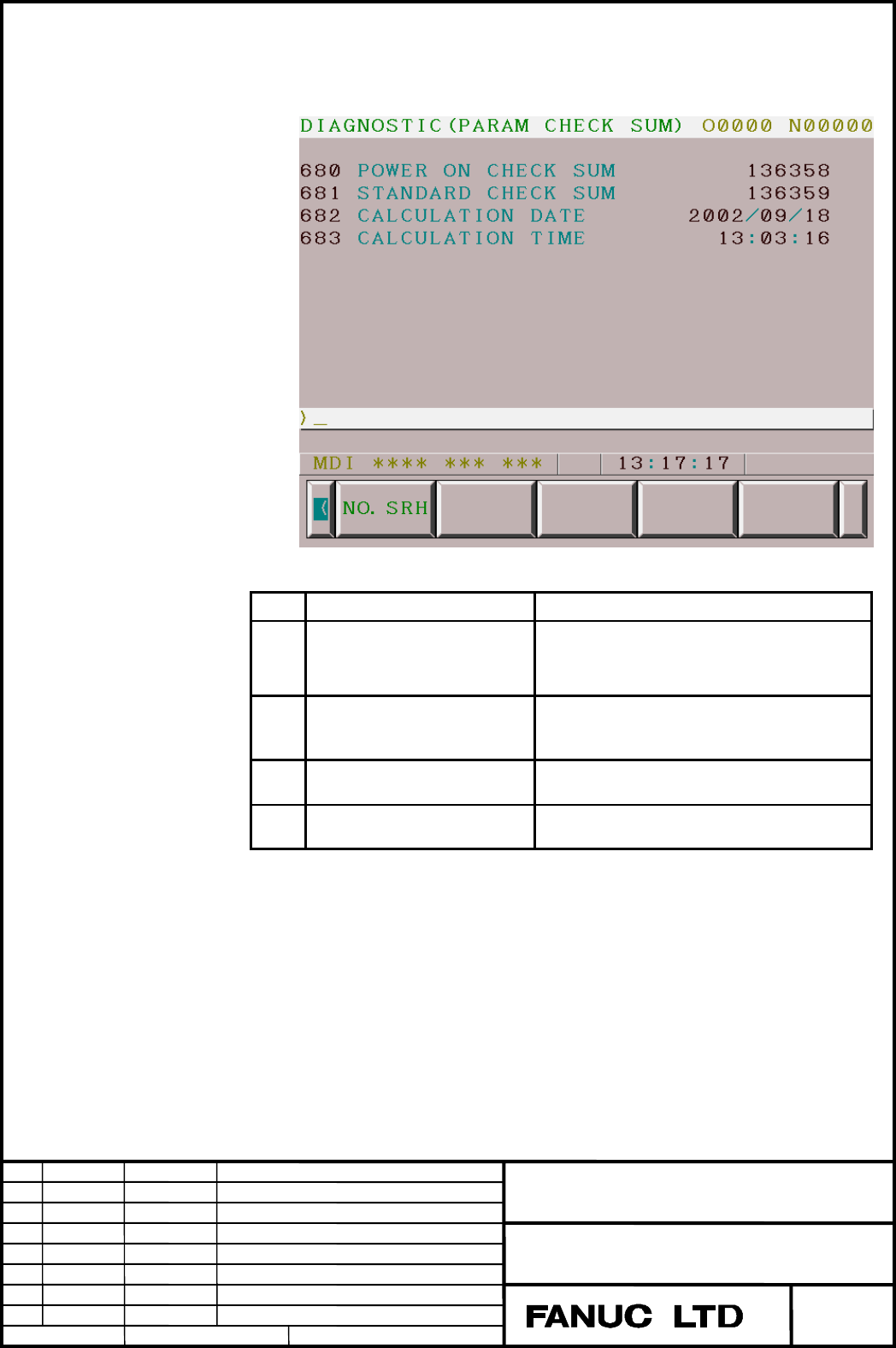# PARAMETER CHECK SUM Function Additional Manual Page 32003/09/05
Edit
EditEdit
Edit
3
/
9
Data
Data Si
g
nature
Si
g
natureSi
g
nature
Si
g
nature
Descri
p
tion
Descri
p
tionDescri
p
tion
Descri
p
tion
FANUC Series 16i/18i/21i-MB
Parameter check sum function Specifications
A
-79242EN
Drawing No.
Title
Page
The power on check sum, the standard check sum, the calculation
date and the calculation time are displayed in the diagnosis screen.
No. Message Meaning of data
680 POWER ON CHECK SUM The parameter check sum which is calculated
at power-on when parameter CKS (No. 13730
# 0) is set to "1" is displayed.
681 STANDARD CHECK SUM The check sum which is calculated when
parameter CKS (No. 13730 # 0) is changed
from "0" to "1" is displayed.
682 CALCULATION DATE The date when the standard check sum is
calculated is displayed.
683 CALCULATION TIME The time when the standard check sum is
calculated is displayed.

#### Contents Summary of PARAMETER CHECK SUM Function Additional Manual

• Page 1FANUC Series 16i/18i/21i-MB Parameter check sum function Specifications Contents 1 GENERAL................................ GENERAL ................................................................ ................................................................................................ .......
• Page 2Parameter check sum function 1 General This function calculates and keeps the standard check sum of CNC parameters. And it also calculates a check sum at CNC power-on, and check whether it equals to the standard check sum or not. 2 Details When parameter CKS (No. 13730 # 0) is changed from ”0” to ”1
• Page 3The power on check sum, the standard check sum, the calculation date and the calculation time are displayed in the diagnosis screen. No. Message Meaning of data 680 POWER ON CHECK SUM The parameter check sum which is calculated at power-on when parameter CKS (No. 13730 # 0) is set to "1" is displaye
• Page 4The check sum is not the sum of all parameters. The following parameters are excluded from the check sum. (1) Setting parameters (2) Parameters shown in the following table (Parameters excluded from the check sum). (3) Parameters specified by parameter No. 13731 to 13750 (4) Parameters specified by
• Page 5In case that the machining condition selecting function is used, parameters in the following table are also excluded from the check sum. Parameters excluded from the check sum (machining condition selecting function) (1/2) Parameter No. Meaning of data 1432 Maximum cutting feed rate for each axis in
• Page 6Parameters excluded from the check sum (machining condition selecting function) (2/2) Parameter No. Meaning of data 19522 Permissible acceleration change level for each axis in speed control based on acceleration changes for jerk control. 19523 Permissible acceleration change level for each axis in
• Page 73 Parameter #7 #6 #5 #4 #3 #2 #1 #0 13730 CSR CKS [Data type] Bit CKS At power-on, checking of the parameter check sum is 0: Not performed. 1: Performed. NOTE When parameter CKS (No. 13730 # 0) is changed from ”0” to ”1”, the standard check sum is calculated. Please cancel all alarms before setting
• Page 813731 Parameter number 1 to exclude from check sum : : 13750 Parameter number 20 to exclude from check sum [Data type] Word [Valid data range ] 0 to +65535 Set parameter number which needs to be excluded from a check sum. 13751 Minimum value 1 of parameter number to exclude from check sum 13752 Maxi
• Page 94 Alarm and message No. Message Meaning of data 5340 PARAMETER CHECK SUM ERROR Because a parameter was modified, the check sum of parameter did not equal to the standard check sum. Please correct parameter value or create the standard check sum again. Title FANUC Series 16i/18i/21i-MB Parameter chec